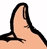Free Space Path Loss

New for May 2008! Let us know if this Rule of Thumb works for you, or if we screwed it up when we edited it.Free space path loss (FSPL) Rule of Thumb, by Stefan from Fraunhofer IZT, an institute that publishes some of the coolest microwave research.

22 dB for the first wavelength,
plus an additional 20xlog(number of wavelengths in the path)

According to Mike, another way of stating the rule is: 22 dB for the first wavelength plus 6 dB for each doubling of distance. Estimate how many doublings of the first wavelength are needed to arrive at the required distance, then multiply this by 6 and add 22. Got that?

Example

Frequency: 1 GHz
Distance: 1 km

Wavelength at 1 GHz is 30 cm. Take 22 dB for the first wavelength.

There's 100 cm in a meter and 1000 meters in a kilometer, so the distance is 100,000 cm

Number of wavelengths for a distance of 1 km:

100,000/30=3300 wavelengths

Now take "normal" dB of 3300

10xlog(3300)=~10xlog(10,000)-10xlog(1/3)=40dB-5dB

(actually, 10xlog of 1/3 is -4.77 dB but this is an approximation, right?)

Because of the 20 log.... the 35 dB becomes 70 dB.

Sum the two contributions to arrive at the estimated path loss at 1 GHz for 1km:

22 dB + 70 dB = 92 dB

With some decibel numbers in memory this estimate can be pretty easy.

Remember:

Factor 2 = 3 dB
Factor 3 = 5 dB (4.77 dB)
Factor 10 = 10 dB

For a more accurate calculation of FSPL, go to our download center and loof for Tim's spreadsheet on this topic.

Author : Unknown Editor